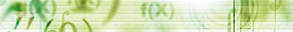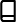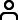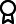Journal metrics
See full report
Acceptance rate24%
Submission to final decision76 days
Acceptance to publication21 days
CiteScore2.200
Journal Citation Indicator0.520
Impact Factor-

### On Hilfer-Type Fractional Impulsive Differential Equations

####Journal profile

International Journal of Differential Equations publishes research on differential equations, and related integral equations, from all scientists who use differential equations as tools within their own discipline.

####Editor spotlight

International Journal of Differential Equations maintains an Editorial Board of practicing researchers from around the world, to ensure manuscripts are handled by editors who are experts in the field of study.

####Special Issues

Do you think there is an emerging area of research that really needs to be highlighted? Or an existing research area that has been overlooked or would benefit from deeper investigation? Raise the profile of a research area by leading a Special Issue.

## Latest Articles

More articles
Research Article

### Positive Invertibility of Matrices and Exponential Stability of Linear Stochastic Systems with Delay

The work addresses the exponential moment stability of solutions of large systems of linear differential Itô equations with variable delays by means of a modified regularization method, which can be viewed as an alternative to the technique based on Lyapunov or Lyapunov-like functionals. The regularization method utilizes the parallelism between Lyapunov stability and input-to-state stability, which is well established in the deterministic case, but less known for stochastic differential equations. In its practical implementation, the method is based on seeking an auxiliary equation, which is used to regularize the equation to be studied. In the final step, estimation of the norm of an integral operator or verification of the property of positivity of solutions is performed. In the latter case, one applies the theory of positive invertible matrices. This report contains a systematic presentation of how the regularization method can be applied to stability analysis of linear stochastic delay equations with random coefficients and random initial conditions. Several stability results in terms of positive invertibility of certain matrices constructed for general stochastic systems with delay are obtained. A number of verifiable sufficient conditions for the exponential moment stability of solutions in terms of the coefficients for specific classes of Itô equations are offered as well.

Research Article

### Solitary Wave Solutions of Nonlinear Integro-Partial Differential Equations of -Dimensional and Its Models

The findings indicate an application of a new method of expansion of the forms and to determine the solutions for wave of the solitary nature in the -dimensional modified form for nonlinear integro-partial differential equations. By using this strategy, we acquired solutions of wave which has a solitary nature that have been solved for three different kinds: hyperbolic, trigonometric, and rational functions. As a result, we obtained different forms of solutions which are new, effective, and powerful to illustrate the solitary nature of waves. The physical and geometrical interpretations have been shown using software in 2 and 3-dimensional surfaces. The obtained results have applications in mathematical and applied sciences. It can also solve different nonlinear integro-partial differential equations which have different applications in physical phenomena using this new method. It has many applications to solve the nonlinear nature of the physical world.

Research Article

### On -Solutions of Some Nonlinear Integral Equations on the Plane

In this paper, we define the space of functions -bounded variation on the plane and endow it with a norm under which it is a Banach space. In addition, we study some nonlinear integral equations and providing conditions for the functions and kernel involved in such equations under which we guarantee the existence and uniqueness in the space of functions of bounded variation in the sense of Shiba on the plane, .

Research Article

### Oscillatory Behavior of Even-Order Half-Linear Neutral Differential Equations

This paper discusses some sufficient conditions for oscillatory behavior of even-order half-linear neutral differential equation. An example is given to illustrate the main result.

Research Article

### Ground State Solutions for a Nonlocal System in Fractional Orlicz-Sobolev Spaces

We consider an elliptic system driven by the fractional -Laplacian operator, with Dirichlet boundary conditions type. By using the Nehari manifold approach, we get a nontrivial ground state solution on fractional Orlicz–Sobolev spaces.

Research Article

### Finite Volume Method for a Time-Dependent Convection-Diffusion-Reaction Equation with Small Parameters

Convection, diffusion, and reaction mechanisms are characteristics of transient mass-transfer phenomena that occur in natural and industrial systems. In this article, we contemplate a passive scalar transport governed by the convection-diffusion-reaction (CDR) equation in 2D flow. The efficiency of solving computationally partial differential equations can be illustrated by using a precise numerical method that yields remarkable precision at a low cost. The accuracy and computational efficiency of two second-order finite difference methods were investigated. The results were compared to a finite volume technique, which has a memory advantage and conserves mass, momentum, and energy even on coarse grids. For various diffusion coefficient values, numerical simulation of unsteady CDR equation are also performed. The techniques were examined for consistency and convergence. The effectiveness and accuracy of these approaches for solving CDR equations are demonstrated by simulation results. Efficiency is measured using and , and the estimated results are compared to the corresponding analytical solution.Journal metrics
See full report
Acceptance rate24%
Submission to final decision76 days
Acceptance to publication21 days
CiteScore2.200
Journal Citation Indicator0.520
Impact Factor-Author guidelinesEditorial boardDatabases and indexingAuthor guidelinesEditorial boardDatabases and indexing

Article of the Year Award: Outstanding research contributions of 2021, as selected by our Chief Editors. Read the winning articles.